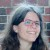# Prem and Ram invested a total of 2,80,000 rupees in a business in the proportion 4:3respectively. What amount did each of th

Prem and Ram invested a total of 2,80,000 rupees in a business in the proportion 4:3
respectively. What amount did each of them invest? If their total profit 2,800 rupees. What
is the share of each?​

### 1 thought on “Prem and Ram invested a total of 2,80,000 rupees in a business in the proportion 4:3<br />respectively. What amount did each of th”

1.Prem) 1,60,000

Ram) 1,20,000

Step-by-step explanation:

$$\frac{280000}{(4 + 3)} \\ = \frac{280000}{7} \\ = 40000$$

Now, Ratio

Prem) 40,000×4=1,60,000

Ram) 40,000×3=1,20,000

SHARE IN PROFIT WOULD BE

$$\frac{2800}{(4 + 3)} \\ = \frac{2800}{7} \\ = 400$$

Now, Ratio

Prem) 400×4=1,600

Ram) 400×3=1,200